# Introduction

Welcome to the StratifiedMedicine R package. The overall goal of this package is to develop analytic and visualization tools to aid in stratified and personalized medicine. Stratified medicine aims to find subsets or subgroups of patients with similar treatment effects, for example responders vs non-responders, while personalized medicine aims to understand treatment effects at the individual level (does a specific individual respond to treatment A?).

Currently, the main tools in this package area: (1) Filter Models (identify important variables and reduce input covariate space), (2) Patient-Level Estimate Models (using regression models, estimate counterfactual quantities, such as the individual treatment effect), (3) Subgroup Models (identify groups of patients using tree-based approaches), and (4) Parameter Estimation (across the identified subgroups), and (5) PRISM (Patient Response Identifiers for Stratified Medicine; combines tools 1-4). Development of this package is ongoing.

As a running example, consider a continuous outcome (ex: % change in tumor size) with a binary treatment (study drug vs standard of care). The estimand of interest is the average treatment effect, $$\theta_0 = E(Y|A=1)-E(Y|A=0)$$. First, we simulate continuous data where roughly 30% of the patients receive no treatment-benefit for using $$A=1$$ vs $$A=0$$. Responders vs non-responders are defined by the continuous predictive covariates $$X_1$$ and $$X_2$$ for a total of four subgroups. Subgroup treatment effects are: $$\theta_{1} = 0$$ ($$X_1 \leq 0, X_2 \leq 0$$), $$\theta_{2} = 0.25 (X_1 > 0, X_2 \leq 0)$$, $$\theta_{3} = 0.45 (X_1 \leq 0, X2 > 0$$), $$\theta_{4} = 0.65 (X_1>0, X_2>0)$$.

library(ggplot2)
library(dplyr)
library(partykit)
library(StratifiedMedicine)
library(survival)
dat_ctns = generate_subgrp_data(family="gaussian")
Y = dat_ctns$Y X = dat_ctns$X # 50 covariates, 46 are noise variables, X1 and X2 are truly predictive
A = dat_ctns$A # binary treatment, 1:1 randomized length(Y) #>  800 table(A) #> A #> 0 1 #> 409 391 dim(X) #>  800 50 # Filter Models The aim of filter models is to potentially reduce the covariate space such that subsequent analyses focus on the “important” variables. For example, we may want to identify variables that are prognostic and/or predictive of the outcome across treatment levels. Filter models can be run using the “filter_train” function. The default is search for prognostic variables using elastic net (Y~ENET(X); Hou and Hastie 2005). Random forest based importance (filter=“ranger”) is also available. See below for an example. Note that the object “filter.vars” contains the variables that pass the filter, while “plot_importance” shows us the relative importance of the input variables. For glmnet, this corresponds to the standardized regression coefficients (variables with coefficients=0 are not shown). res_f <- filter_train(Y, A, X, filter="glmnet") res_f$filter.vars
#>   "X1"  "X2"  "X3"  "X5"  "X7"  "X8"  "X10" "X12" "X16" "X18" "X24" "X26"
#>  "X31" "X40" "X46" "X50"
plot_importance(res_f)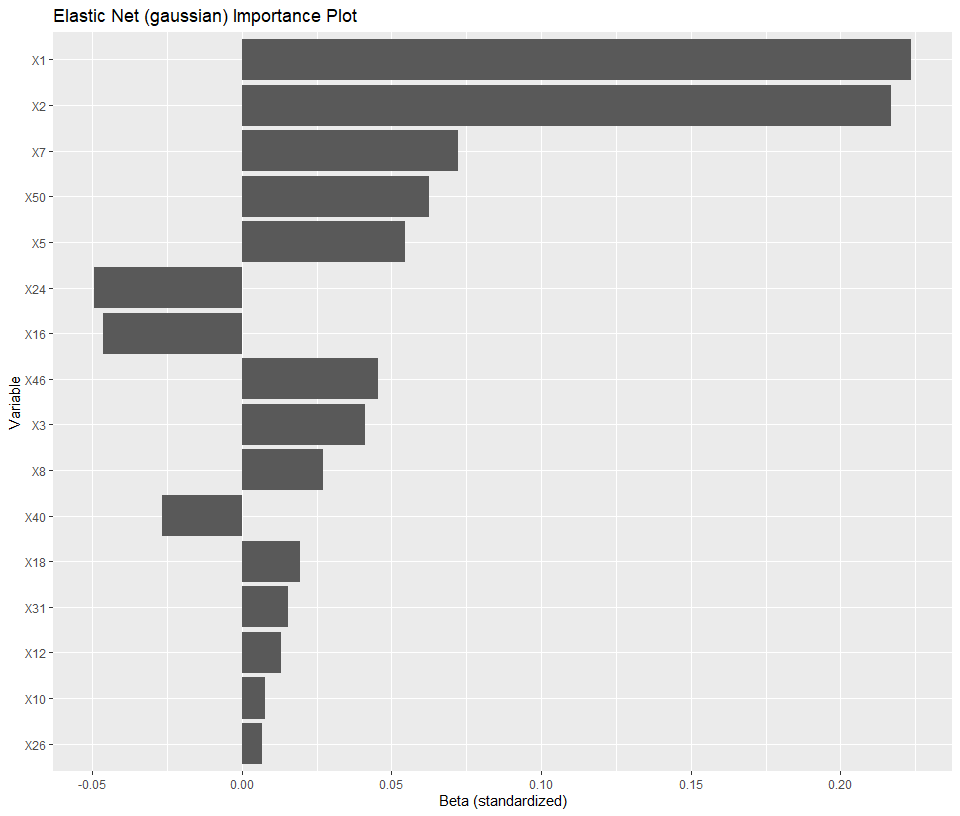An alternative approach is to search for variables that are potentially prognostic and/or predictive by forcing variable by treatment interactions, or Y~ENET(A,X,XA). Variables with estimated coefficients of 0 in both the main effects (X) and interaction effects (XA) are filtered. This can be implemented by tweaking the hyper-parameters:

res_f2 <- filter_train(Y, A, X, filter="glmnet", hyper=list(interaction=T))
res_f2$filter.vars #>  "X1" "X2" "X3" "X5" "X7" "X8" "X12" "X16" "X18" "X23" "X24" "X26" #>  "X31" "X46" "X50" "X10" "X17" "X20" "X37" "X39" "X40" plot_importance(res_f2)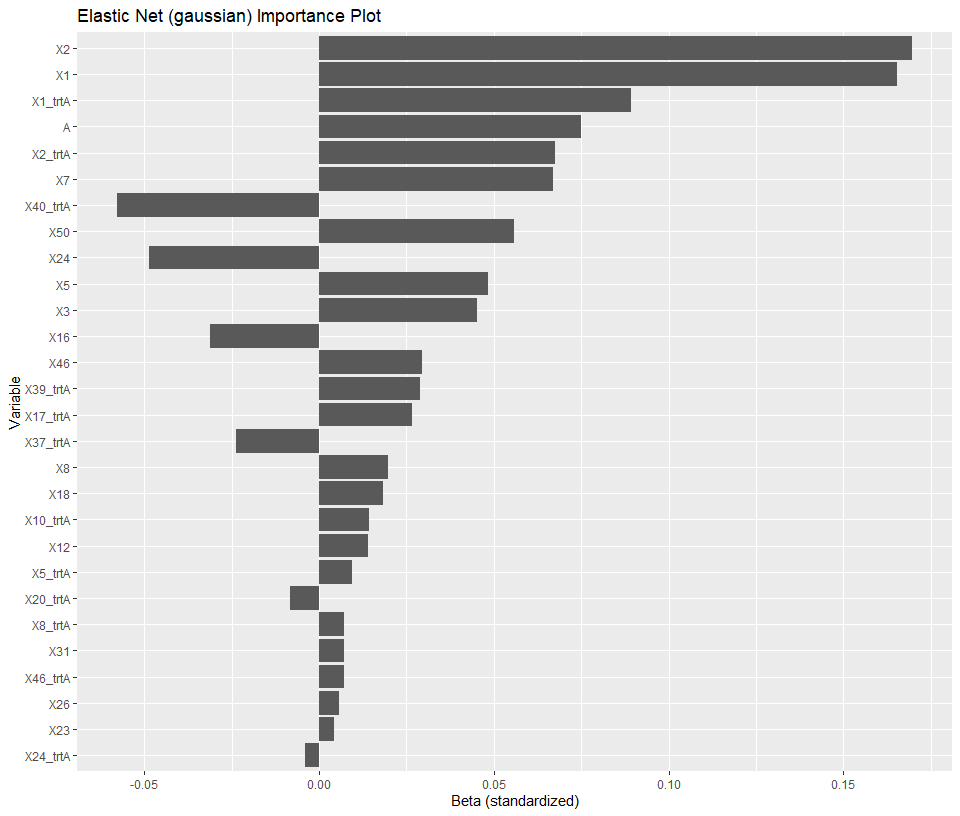Here, note that both the main effects of X1 and X2, along with the interaction effects (labeled X1_trtA and X2_trtA), have relatively large estimated coefficients. # Patient-level Estimate (PLE) Models The aim of PLE models is to estimate counterfactual quantities, for example the individual treatment effect. This is implemented through the “ple_train” function. The “ple_train” follows the framework of Kunzel et al 2019, which utilizes base learners and meta learners to obtain estimates of interest. For family=“gaussian”, “binomial”, this output estimates of and treatment differences. For family=“survival”, either logHR or restricted mean survival time (RMST) estimates are obtained. Current base-leaner options include “linear” (lm/glm/or cox), “ranger” (random forest through ranger R package), “glmnet” (elastic net), and “bart” (Bayesian Additive Regression Trees through BART R package). Meta-learners include the “T-Leaner” (treatment specific models), “S-learner” (single regression model), and “X-learner” (2-stage approach, see Kunzel et al 2019). See below for an example. Note that the object “mu_train” contains the training set patient-level estimates (outcome-based and propensity scores), “plot_ple” shows a waterfall plot of the estimated individual treatment effects, and “plot_dependence” shows the partial dependence plot for variable “X1” with respect to the estimated individual treatment effect. res_p1 <- ple_train(Y, A, X, ple="ranger", meta="X-learner") summary(res_p1$mu_train)
#>       mu_0             mu_1           diff_1_0             pi_0
#>  Min.   :0.6493   Min.   :0.4634   Min.   :-0.38289   Min.   :0.5112
#>  1st Qu.:1.4165   1st Qu.:1.5749   1st Qu.: 0.09688   1st Qu.:0.5112
#>  Median :1.6220   Median :1.8047   Median : 0.20678   Median :0.5112
#>  Mean   :1.6339   Mean   :1.8435   Mean   : 0.20895   Mean   :0.5112
#>  3rd Qu.:1.8417   3rd Qu.:2.1031   3rd Qu.: 0.31797   3rd Qu.:0.5112
#>  Max.   :2.6318   Max.   :3.1174   Max.   : 0.70128   Max.   :0.5112
#>       pi_1
#>  Min.   :0.4888
#>  1st Qu.:0.4888
#>  Median :0.4888
#>  Mean   :0.4888
#>  3rd Qu.:0.4888
#>  Max.   :0.4888
plot_ple(res_p1, target = "diff_1_0") +
ggtitle("Waterfall Plot: E(Y|A=1)-E(Y|A=0)") + ylab("E(Y|A=1)-E(Y|A=0)")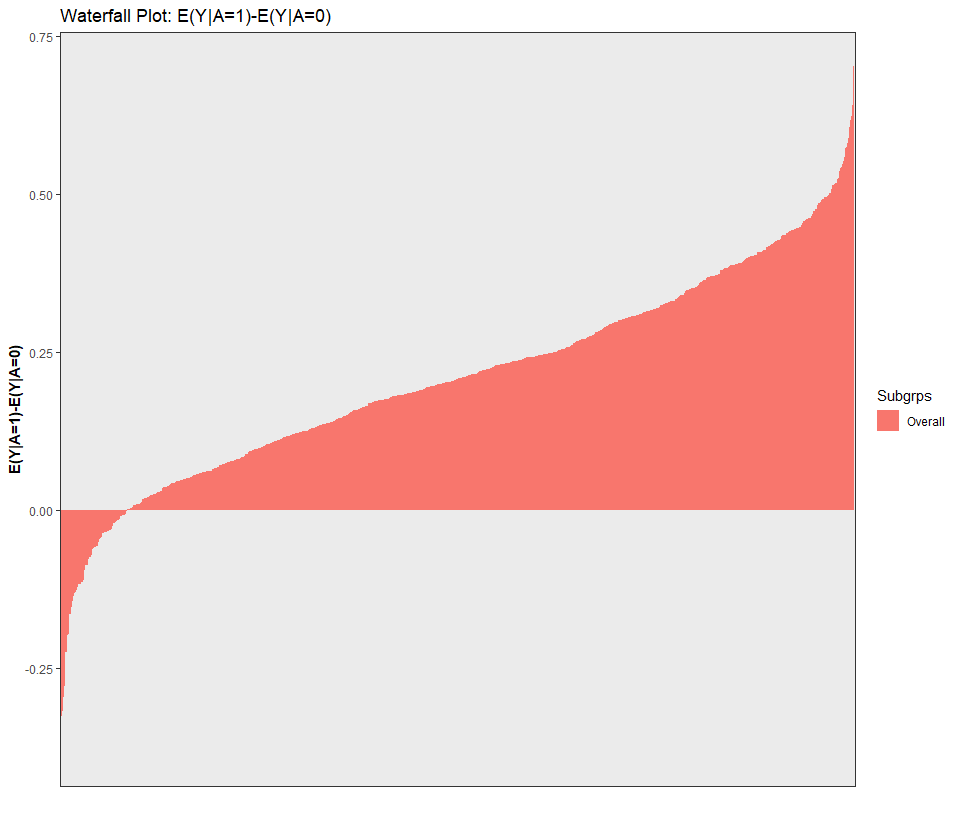plot_dependence(res_p1, X=X, vars="X1") + ylab("E(Y|A=1)-E(Y|A=0)")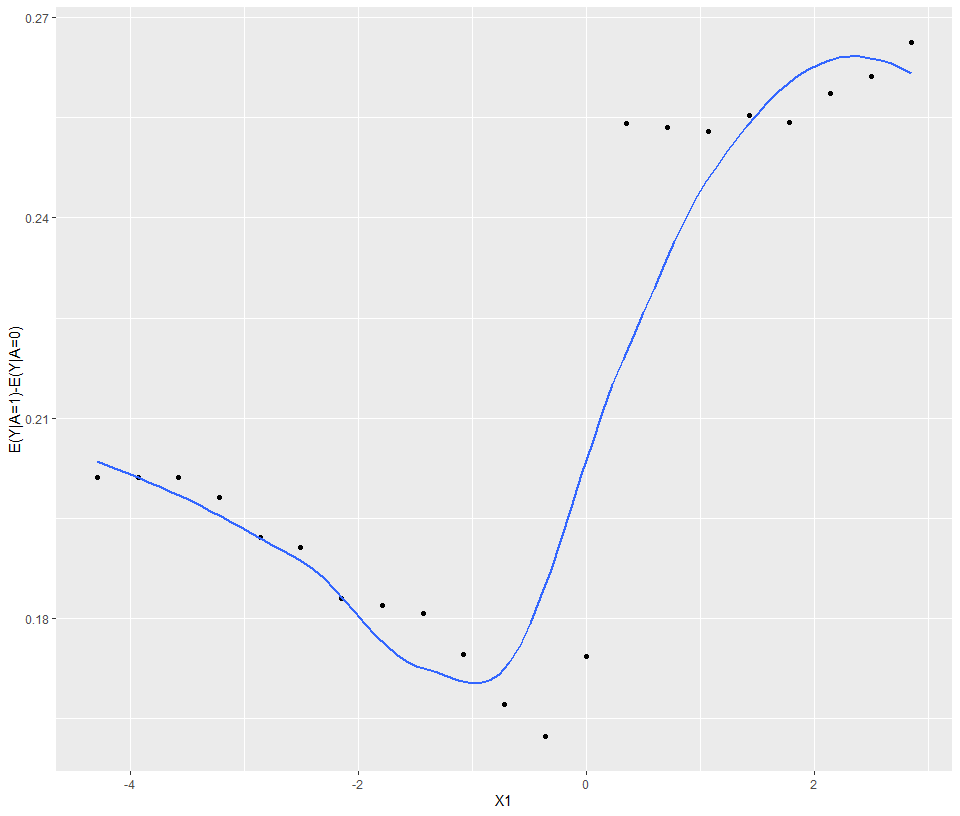Next, let’s illustrate how to change the meta-learner and the hyper-parameters. See below, along with a 2-dimension PDP plot.

res_p2 <- ple_train(Y, A, X, ple="ranger", meta="T-learner", hyper=list(mtry=5))
summary(res_p2$mu_train) #> mu_0 mu_1 diff_1_0 pi_0 #> Min. :0.7052 Min. :0.5131 Min. :-1.0249 Min. :0.5112 #> 1st Qu.:1.4341 1st Qu.:1.6060 1st Qu.:-0.0202 1st Qu.:0.5112 #> Median :1.6323 Median :1.8196 Median : 0.2125 Median :0.5112 #> Mean :1.6398 Mean :1.8457 Mean : 0.2059 Mean :0.5112 #> 3rd Qu.:1.8451 3rd Qu.:2.0851 3rd Qu.: 0.4443 3rd Qu.:0.5112 #> Max. :2.5934 Max. :2.9993 Max. : 1.0341 Max. :0.5112 #> pi_1 #> Min. :0.4888 #> 1st Qu.:0.4888 #> Median :0.4888 #> Mean :0.4888 #> 3rd Qu.:0.4888 #> Max. :0.4888 plot_ple(res_p2, target = "diff_1_0") + ggtitle("Waterfall Plot: E(Y|A=1)-E(Y|A=0)") + ylab("E(Y|A=1)-E(Y|A=0)")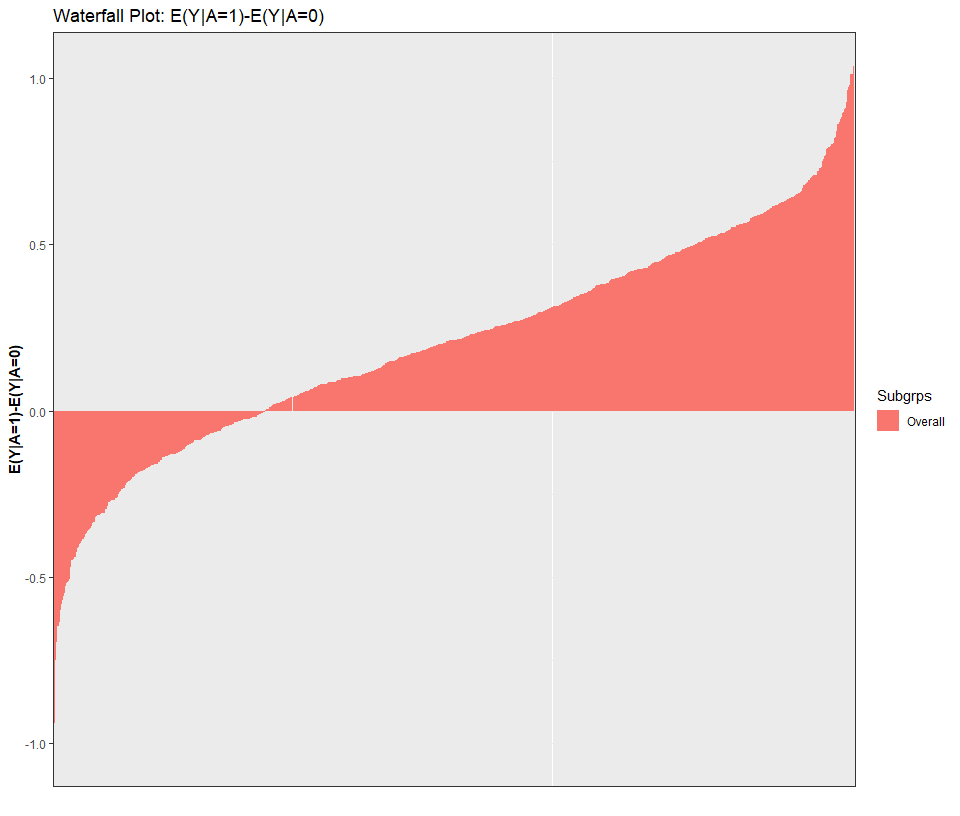plot_dependence(res_p2, X=X, vars=c("X1", "X2")) + ggtitle("Heat Map (By X1,X2): E(Y|A=1)-E(Y|A=0)")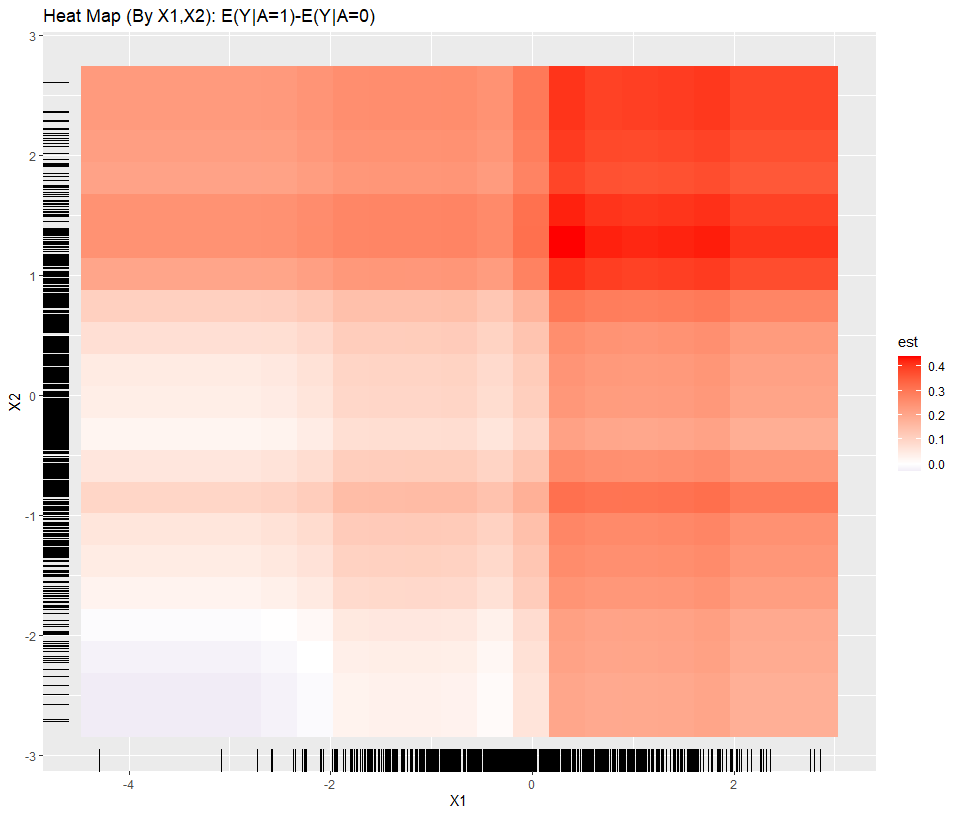ylab("E(Y|A=1)-E(Y|A=0)") #>$y
#>  "E(Y|A=1)-E(Y|A=0)"
#>
#> attr(,"class")
#>  "labels"

# Subgroup Models

Subgroup models are called using the “submod_train” function and currently only include tree-based methods (ctree, lmtree, glmtree from partykit R package and rpart from rpart R package). First, let’s run the default (for continuous, uses lmtree). This aims to find subgroups that are either prognostic and/or predictive.

res_s1 <- submod_train(Y, A, X, submod="lmtree")
table(res_s1$Subgrps.train) #> #> 3 4 6 7 #> 149 277 267 107 plot(res_s1$fit$mod)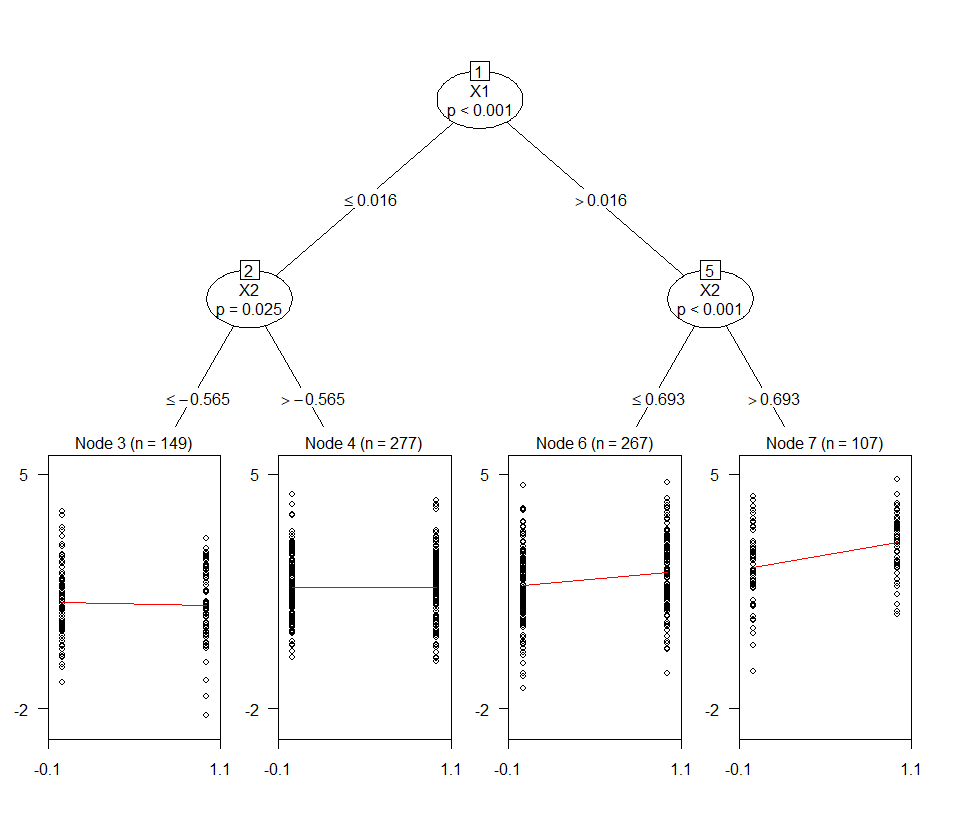Another generic approach is “otr”, which follows an outcome weighted learning approach. Here, we regress PLE ~ ctree(X) with weights=abs(PLE) where PLE=E(Y|A=1,X)-E(Y|A=0,X) is the estimated individual treatment effect. For survival endpoints, the treatment difference would correspond to either logHR or RMST. For the example below, we set the clinically meaningful threshold to 0.1 (thres=“>0.10”). res_s2 <- submod_train(Y, A, X, thres = ">0.10", mu_train=res_p2$mu_train, submod="otr")
#>    Min. 1st Qu.  Median    Mean 3rd Qu.    Max.
#> -1.0249 -0.0202  0.2125  0.2059  0.4443  1.0341
plot(res_s2$fit$mod)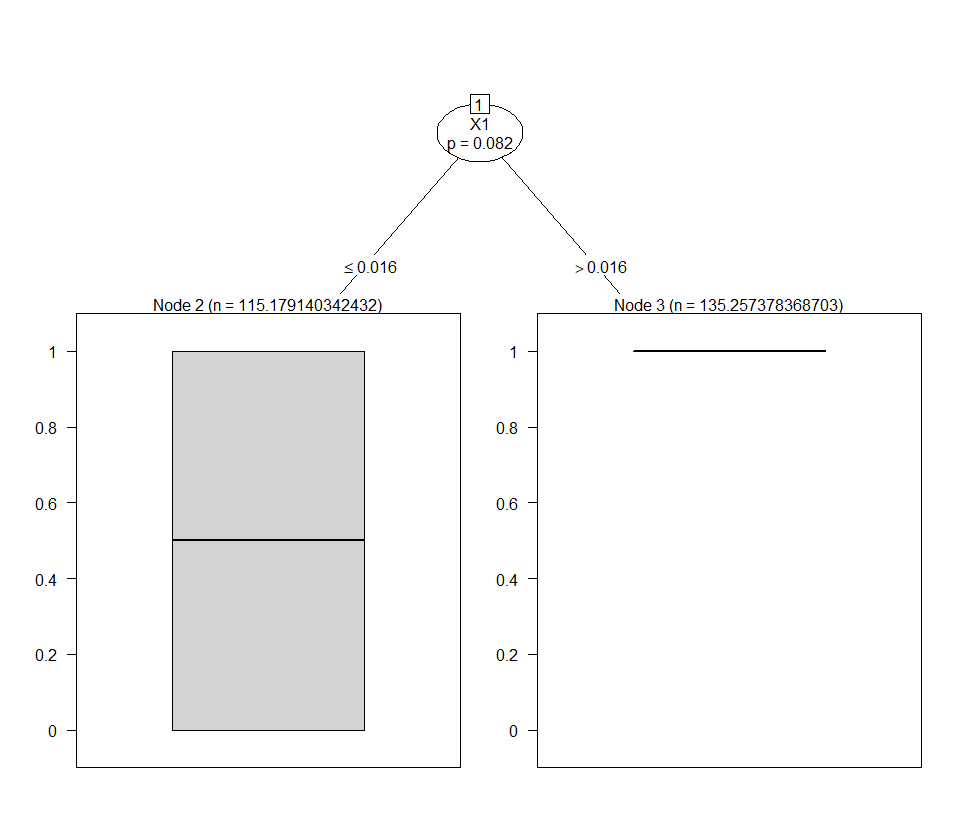# Parameter Estimation

To facilitate parameter estimation across the identified subgroups, “StratifiedMedicine” currently includes the function “param_est.” This includes param=“lm”, “dr”, “ple”, “cox”, and “rmst” which correspond respectively to linear regression, the doubly robust estimator, average the patient-level estimates, cox regresson, and RMST (as in survRM2 R package). Notably, if the subgroups are determined adaptively (for example through lmtree), without resampling corrections, point-estimates tend to be overly optimistic. We address this later.

Given a candidate set of subgroups, a simple approach is to fit linear regression models within each subgroup to obtain treatment-specific and treatment-difference estimates. See below.

param.dat1 <- param_est(Y, A, X, Subgrps = res_s1$Subgrps.train, param="lm") param.dat1 %>% filter(estimand=="mu_1-mu_0") #> Subgrps N estimand est SE LCL UCL #> 1 ovrl 800 mu_1-mu_0 0.214721873 0.07270356 0.07200932 0.3574344 #> 2 3 149 mu_1-mu_0 -0.074540934 0.17749849 -0.42529970 0.2762178 #> 3 4 277 mu_1-mu_0 -0.002507699 0.11778878 -0.23438626 0.2293709 #> 4 6 267 mu_1-mu_0 0.392978208 0.12852946 0.13991368 0.6460427 #> 5 7 107 mu_1-mu_0 0.735079896 0.19631153 0.34587320 1.1242866 #> pval alpha #> 1 0.0032352410 0.05 #> 2 0.6751291856 0.05 #> 3 0.9830298696 0.05 #> 4 0.0024593995 0.05 #> 5 0.0002942317 0.05 Alternatively, we may instead use the doubly-robust estimator, which combines the observed outcome (Y) and model estimates from “ple_train”. This requires inputting model estimates (see “mu_hat”). See below: param.dat2 <- param_est(Y, A, X, Subgrps = res_s1$Subgrps.train,
mu_hat = res_p1$mu_train, param="dr") param.dat2 %>% filter(estimand=="mu_1-mu_0") #> Subgrps N estimand est SE LCL UCL #> 1 ovrl 800 mu_1-mu_0 0.20958932 0.06361335 0.08472028 0.3344583 #> 2 3 149 mu_1-mu_0 -0.04117054 0.15841845 -0.35422480 0.2718837 #> 3 4 277 mu_1-mu_0 0.02191513 0.10010936 -0.17515979 0.2189901 #> 4 6 267 mu_1-mu_0 0.35692736 0.10860576 0.14309105 0.5707637 #> 5 7 107 mu_1-mu_0 0.67696977 0.18087503 0.31836743 1.0355721 #> pval alpha #> 1 0.0010285354 0.05 #> 2 0.7953138521 0.05 #> 3 0.8268804462 0.05 #> 4 0.0011509845 0.05 #> 5 0.0002960023 0.05 # PRISM: Patient Response Identifiers for Stratified Medicine While the above tools individually can be useful, PRISM (Patient Response Identifiers for Stratified Medicine; Jemielita and Mehrotra (to appear), https://arxiv.org/abs/1912.03337) combines each component for a stream-lined analysis. Given a data-structure of $$(Y, A, X)$$ (outcome(s), treatments, covariates), PRISM is a five step procedure: 1. Estimand: Determine the question(s) or estimand(s) of interest. For example, $$\theta_0 = E(Y|A=1)-E(Y|A=0)$$, where A is a binary treatment variable. While this isn’t an explicit step in the PRISM function, the question of interest guides how to set up PRISM. 2. Filter (filter): Reduce covariate space by removing variables unrelated to outcome/treatment. 3. Patient-level estimate (ple): Estimate counterfactual patient-level quantities, for example the individual treatment effect, $$\theta(x) = E(Y|X=x,A=1)-E(Y|X=x,A=0)$$. These can be used in the subgroup model and/or parameter estimation. 4. Subgroup model (submod): Identify subgroups of patients with potentially varying treatment response. 5. Parameter estimation and inference (param): For the overall population and discovered subgroups, output point estimates and variability metrics. If the subgroups are determined adaptively, resampling is needed to avoid overly optimistic point estimates and to form CIs. 6. Resampling: Repeat Steps 1-4 across $$R$$ non-parametric bootstrap resamplings to generate subgroup-specific parameter estimate bootstrap distributions. Ultimately, PRISM provides information at the patient-level, the subgroup-level (if any), and the overall population. While there are defaults in place, the user can also input their own functions/model wrappers into the PRISM algorithm. We will demonstrate this later. PRISM can also be run without treatment assignment (A=NULL); in this setting, the focus is on finding subgroups based on prognostic effects. The below table describes default PRISM configurations for different family (gaussian, biomial, survival) and treatment (no treatment vs treatment) settings, including the associated estimands. Note that OLS refers to ordinary least squares (linear regression), GLM refers to generalized linear model, and MOB refers to model based partitioning (Zeileis, Hothorn, Hornik 2008; Seibold, Zeileis, Hothorn 2016). To summarise, default models include elastic net (Zou and Hastie 2005) for filtering, random forest (“ranger” R package) for patient-level /counterfactual estimation, and MOB (through “partykit” R package; lmtree, glmtree, and ctree (Hothorn, Hornik, Zeileis 2005)). When treatment assignment is provided, parameter estimation for continuous and binary outcomes involves averaging the patient-level estimates within the overall population and discovered subgroups (more details later). For survival outcomes, the cox regression hazard ratio (HR) or RMST (from the survR2 package) is used. Default PRISM Configurations (With Treatment) Step gaussian binomial survival estimand(s) E(Y|A=0) E(Y|A=1) E(Y|A=1)-E(Y|A=0) E(Y|A=0) E(Y|A=1) E(Y|A=1)-E(Y|A=0) HR(A=1 vs A=0) filter Elastic Net (glmnet) Elastic Net (glmnet) Elastic Net (glmnet) ple X-learner: Random Forest (ranger) X-learner: Random Forest (ranger) T-learner: Random Forest (ranger) submod MOB(OLS) (lmtree) MOB(GLM) (glmtree) MOB(weibull) (mob_weib) param Double Robust (dr) Doubly Robust (dr) Hazard Ratios (cox) Default PRISM Configurations (Without Treatment, A=NULL) Step gaussian binomial survival estimand(s) E(Y) Prob(Y) RMST filter Elastic Net (glmnet) Elastic Net (glmnet) Elastic Net (glmnet) ple Random Forest (ranger) Random Forest (ranger) Random Forest (ranger) submod Conditional Inference Trees (ctree) Conditional Inference Trees (ctree) Conditional Inference Trees (ctree) param OLS (lm) OLS (lm) RMST (rmst) # Example: Continuous Outcome with Binary Treatment For continuous outcome data (family=“gaussian”), the default PRISM configuration is: (1) filter=“glmnet” (elastic net), (2) ple=“ranger” (X-learner with random forest models), (3) submod=“lmtree” (model-based partitioning with OLS loss), and (4) param=“dr” (doubly-robust estimator). To run PRISM, at a minimum, the outcome (Y), treatment (A), and covariates (X) must be provided. See below. # PRISM Default: filter_glmnet, ranger, lmtree, param_ple # res0 = PRISM(Y=Y, A=A, X=X) #> Observed Data #> Filtering: glmnet #> PLE: ranger #> Subgroup Identification: lmtree #> Parameter Estimation: dr summary(res0) #>$PRISM Configuration
#>  "glmnet => ranger => lmtree => dr"
#>
#> $Variables that Pass Filter #>  "X1" "X2" "X3" "X5" "X7" "X8" "X10" "X12" "X16" "X18" "X24" "X26" #>  "X31" "X40" "X46" "X50" #> #>$Number of Identified Subgroups
#>  6
#>
#> $Variables that Define the Subgroups #>  "X1, X2, X50, X26" #> #>$Parameter Estimates
#>    Subgrps   N  estimand     est     SE alpha               CI
#> 4       10 168      mu_0  1.9265 0.0860  0.05  [1.7567,2.0964]
#> 7       11 107      mu_0  2.1880 0.1332  0.05  [1.9238,2.4521]
#> 10       3 149      mu_0  1.1687 0.1110  0.05   [0.9494,1.388]
#> 13       5 110      mu_0  1.3177 0.1057  0.05  [1.1083,1.5271]
#> 16       6 167      mu_0  1.8470 0.0813  0.05  [1.6865,2.0074]
#> 19       9  99      mu_0  1.2580 0.1491  0.05  [0.9621,1.5539]
#> 1     ovrl 800      mu_0  1.6373 0.0457  0.05   [1.5477,1.727]
#> 5       10 168      mu_1  2.1155 0.1003  0.05  [1.9176,2.3135]
#> 8       11 107      mu_1  2.9055 0.1134  0.05  [2.6808,3.1303]
#> 11       3 149      mu_1  1.1311 0.1092  0.05  [0.9154,1.3469]
#> 14       5 110      mu_1  1.5376 0.1179  0.05  [1.3039,1.7713]
#> 17       6 167      mu_1  1.7067 0.1023  0.05  [1.5047,1.9087]
#> 20       9  99      mu_1  1.8960 0.1184  0.05   [1.661,2.1311]
#> 2     ovrl 800      mu_1  1.8459 0.0487  0.05  [1.7504,1.9414]
#> 6       10 168 mu_1-mu_0  0.1890 0.1311  0.05 [-0.0698,0.4478]
#> 9       11 107 mu_1-mu_0  0.7176 0.1762  0.05  [0.3682,1.0669]
#> 12       3 149 mu_1-mu_0 -0.0376 0.1541  0.05  [-0.3422,0.267]
#> 15       5 110 mu_1-mu_0  0.2199 0.1586  0.05 [-0.0944,0.5343]
#> 18       6 167 mu_1-mu_0 -0.1403 0.1298  0.05 [-0.3967,0.1161]
#> 21       9  99 mu_1-mu_0  0.6380 0.1880  0.05  [0.2649,1.0112]
#> 3     ovrl 800 mu_1-mu_0  0.2086 0.0633  0.05  [0.0843,0.3328]
#>
#> attr(,"class")
#>  "summary.PRISM"
plot(res0) # same as plot(res0, type="tree")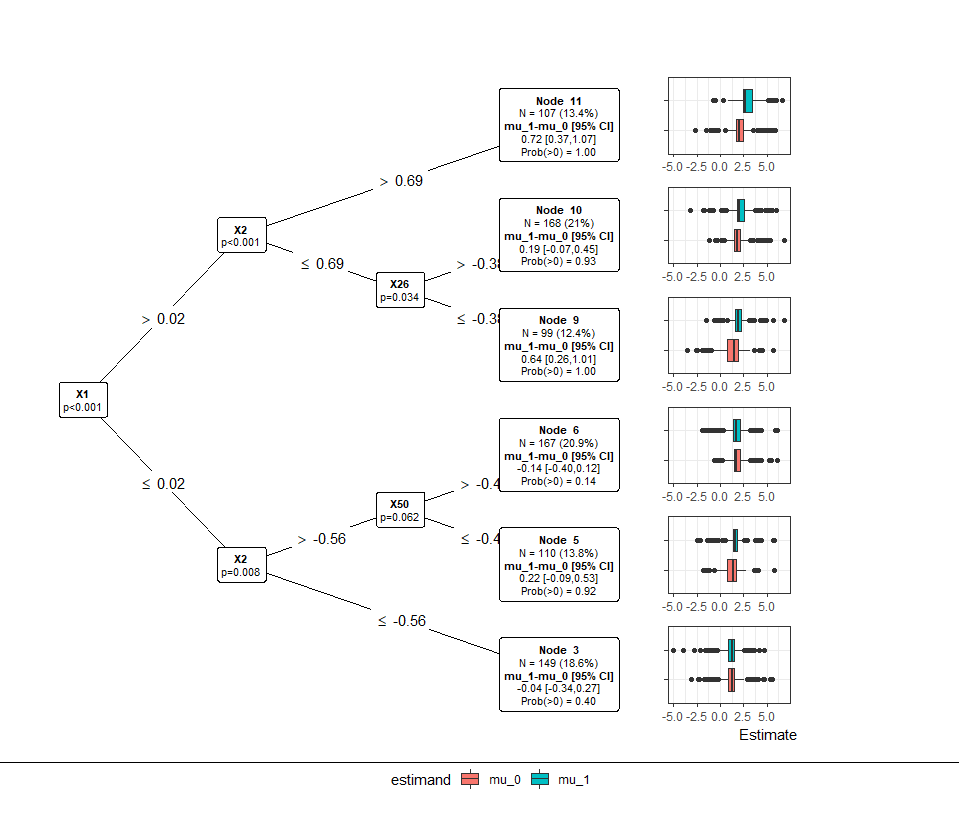The summary gives a high-level overview of the findings (number of subgroups, parameter estimates, variables that survived the filter). The default plot() function currently combines tree plots with parameter estimates using the “ggparty” package. We can als directly look for prognostic effects by specifying omitting A (treatment) from PRISM:

# PRISM Default: filter_glmnet, ranger, ctree, param_lm #
res_prog = PRISM(Y=Y, X=X)
#> No Treatment Variable (A) Provided: Searching for Prognostic Effects
#> Observed Data
#> Filtering: glmnet
#> PLE: ranger
#> Subgroup Identification: ctree
#> Parameter Estimation: lm
# res_prog = PRISM(Y=Y, A=NULL, X=X) #also works
summary(res_prog)
#> $PRISM Configuration #>  "glmnet => ranger => ctree => lm" #> #>$Variables that Pass Filter
#>   "X1"  "X2"  "X3"  "X5"  "X7"  "X8"  "X10" "X12" "X16" "X18" "X24" "X26"
#>  "X31" "X40" "X46" "X50"
#>
#> $Number of Identified Subgroups #>  6 #> #>$Variables that Define the Subgroups
#>  "X2, X1, X26"
#>
#> $Parameter Estimates #> Subgrps N estimand est SE alpha CI #> 2 10 87 mu 1.9091 0.1006 0.05 [1.7091,2.1091] #> 3 11 80 mu 2.6842 0.1133 0.05 [2.4586,2.9097] #> 4 4 132 mu 1.1119 0.0970 0.05 [0.92,1.3038] #> 5 5 266 mu 1.5107 0.0636 0.05 [1.3855,1.636] #> 6 7 113 mu 1.7016 0.0995 0.05 [1.5045,1.8987] #> 7 8 122 mu 2.1780 0.0856 0.05 [2.0085,2.3474] #> 1 ovrl 800 mu 1.7343 0.0363 0.05 [1.663,1.8056] #> #> attr(,"class") #>  "summary.PRISM" plot(res_prog)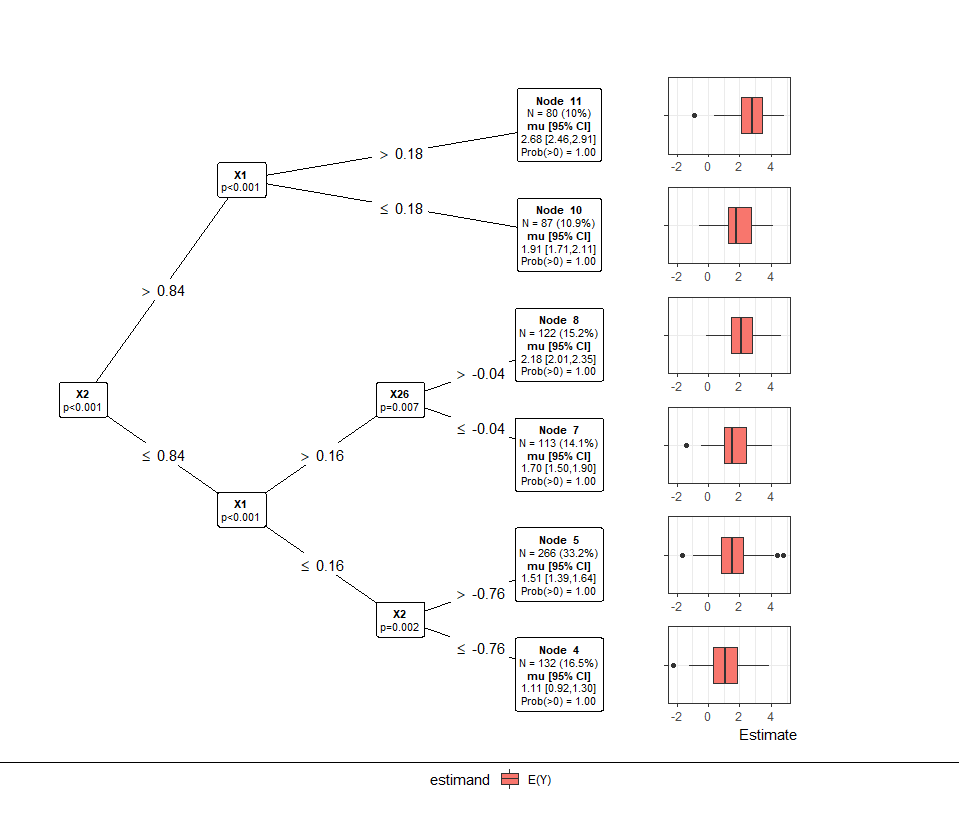Next, circling back to the first PRISM model with treatment included, let’s review other core PRISM outputs. Results relating to the filter include “filter.mod” (model output) and “filter.vars” (variables that pass the filter). The “plot_importance” function can also be called: # elastic net model: loss by lambda # plot(res0$filter.mod)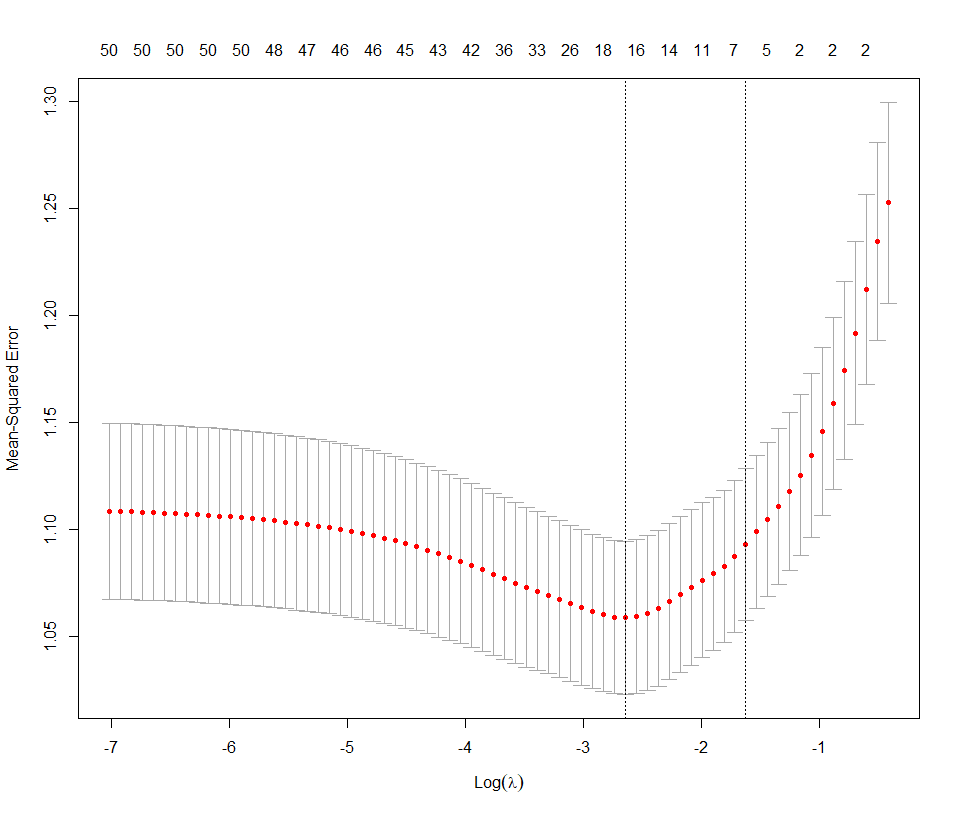## Variables that remain after filtering ##
res0$filter.vars #>  "X1" "X2" "X3" "X5" "X7" "X8" "X10" "X12" "X16" "X18" "X24" "X26" #>  "X31" "X40" "X46" "X50" # All predictive variables (X1,X2) and prognostic variables (X3,X5, X7) remains. plot_importance(res0)Results relating to “ple_train” include “ple.fit” (fitted “ple_train”), “mu.train” (training predictions), and “mu.test” (test predictions). “plot_ple” and “plot_dependence” can also be used with PRISM objects. For example, plot_ple(res0)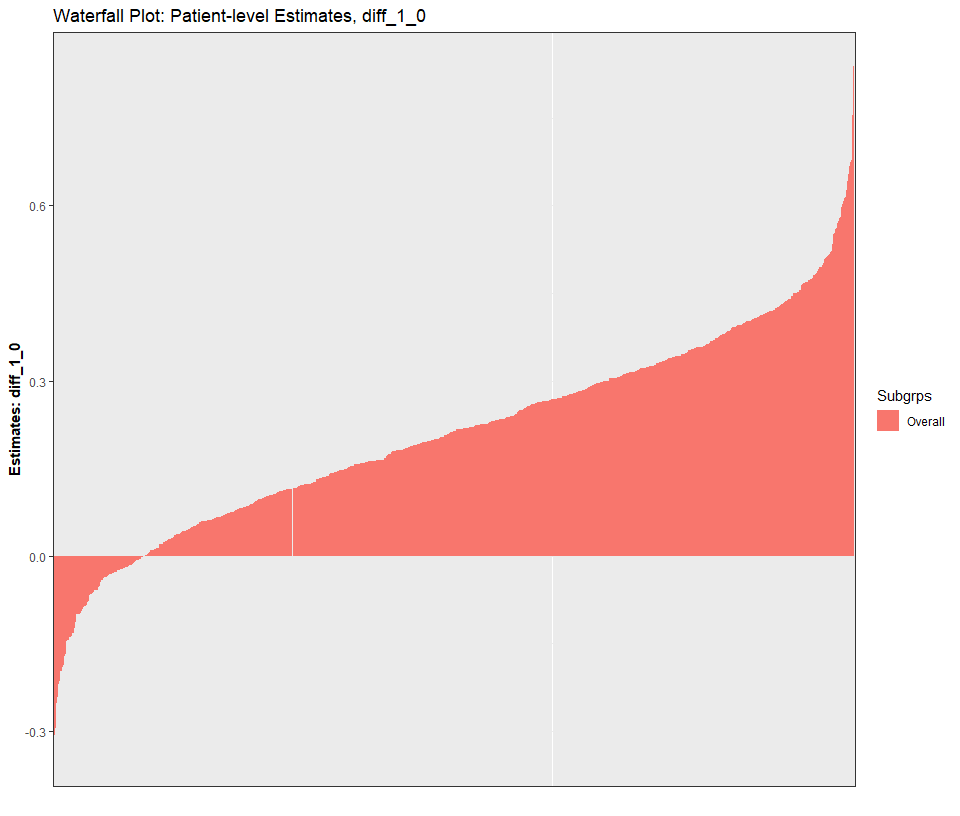plot_dependence(res0, vars=c("X2")) #> geom_smooth() using method = 'loess' and formula 'y ~ x'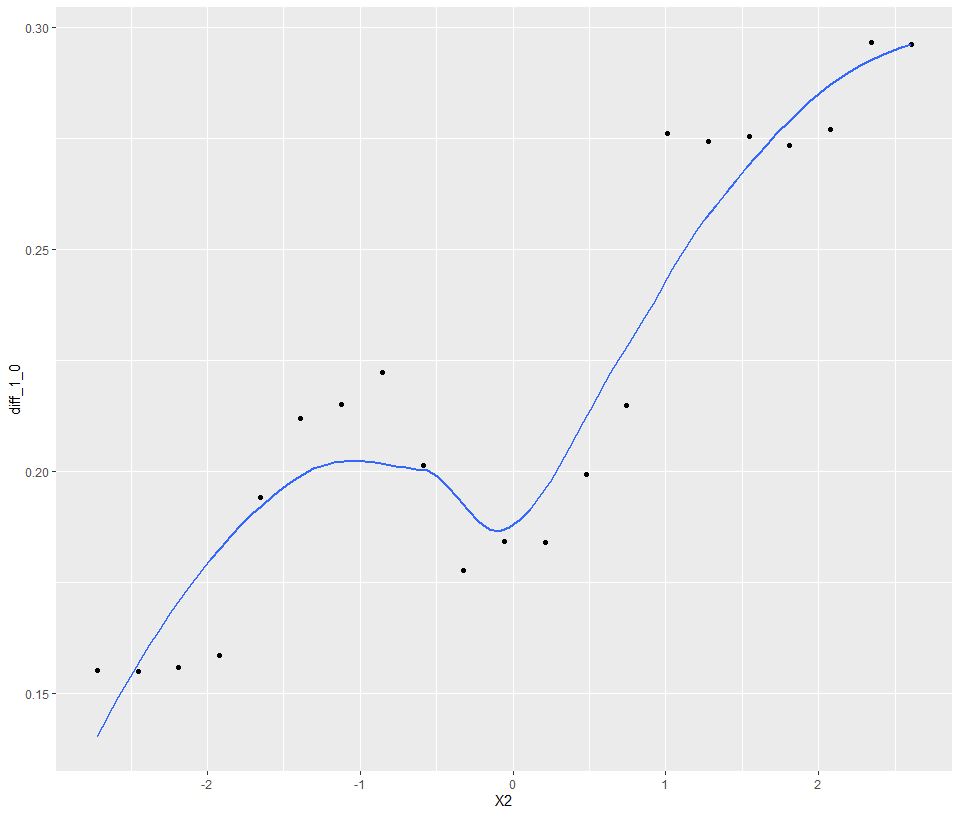Next, the subgroup model (lmtree), identifies 4-subgroups based on varying treatment effects. By plotting the subgroup model object (“submod.fit$mod”)“, we see that partitions are made through X1 (predictive) and X2 (predictive). At each node, parameter estimates for node (subgroup) specific OLS models, $$Y\sim \beta_0+\beta_1*A$$. For example, patients in nodes 4 and 6 have estimated treatment effects of 0.47 and 0.06 respectively. Subgroup predictions for the train/test set can be found in the”out.train" and “out.test” data-sets.

plot(res0$submod.fit$mod, terminal_panel = NULL)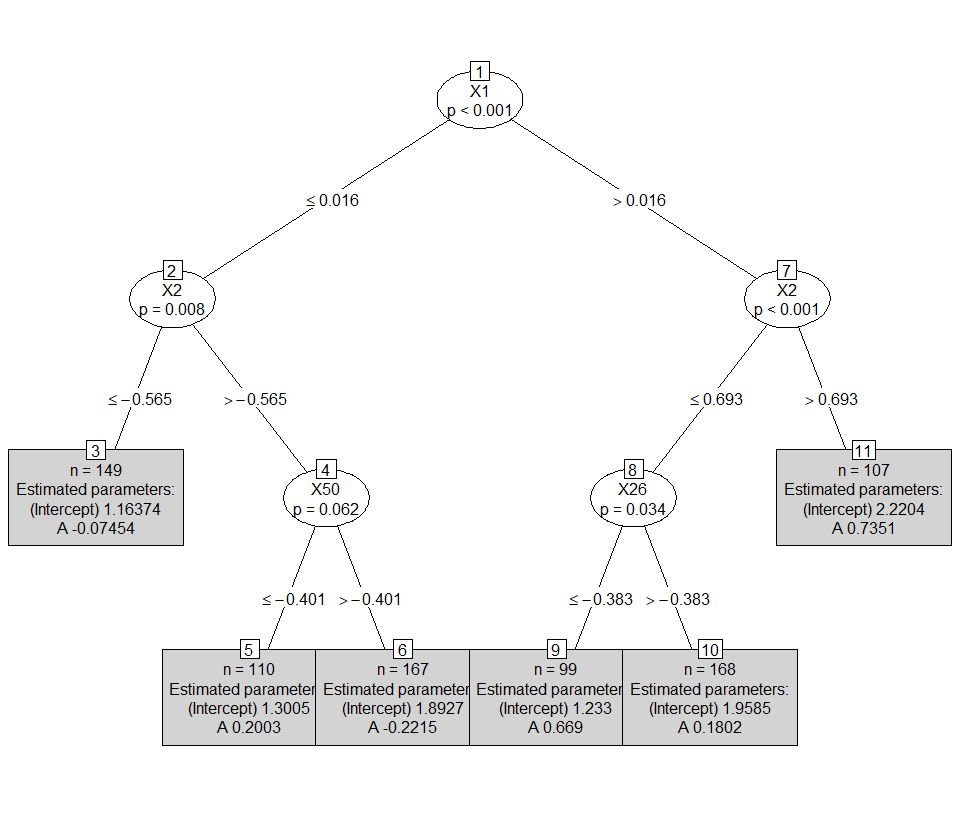table(res0$out.train$Subgrps)
#>
#>  10  11   3   5   6   9
#> 168 107 149 110 167  99
table(res0$out.test$Subgrps)
#>
#>  10  11   3   5   6   9
#> 168 107 149 110 167  99

For any parameter estimation approache, subgroup-specific estimates tend to be overly positive or negative, as the same data that trains the subgroup model is used for parameter estimation. Resampling, such as bootstrapping, is generally perferred for “honest” treatment effect estimates (more details below).

For continuous and binary data, the default parameter estimation approach is param=“dr” (double robust estimator). This approach incorporates regression estimates, which could potentially increase the efficiency of the point-estimate. Let $$k=1,...,K$$ index the $$K$$ identified subgroups with corresponding rules $$S_1,...,S_K$$. Next, let $$E(Y|X=x,A=a) = \mu(x, a)$$ correspond to the outcome regression model(s) with estimates $$\hat{\mu}(x, a)$$. These estimates come directly from the fitted PLE model(s), in this case, treatment-specific random forest models. Define the “pseudo-outcomes” as:

$Y^{\star}_i = \frac{AY - (A-\hat{\pi}(x))\hat{\mu}(a=1,x)}{\hat{\pi}(x)} - \frac{(1-A)Y - (A-\hat{\pi}(x))\hat{\mu}(a=0,x)}{1-\hat{\pi}(x)}$

where $$\pi(x)=P(A=1|X)$$, or the treatment assignment probability for an individual. In a randomized controlled trial, this can be replaced by the marginal probability, $$P(A=1|X)$$. For each discovered subgroup ($$k=1,...,K$$), the treatment effect (or risk difference) and associated SE are then: can be estimated by averaging the patient-specific treatment effect estimates (PLEs): $\hat{\theta}_k = \sum_{i \in S_k} Y^{\star}_i$ $SE(\hat{\theta}_k) = \sqrt{ n_k ^ {-2} \sum_{i \in S_k} \left( Y^{\star}_i-\hat{\theta}(x_i) \right)^2}$ CIs can then be formed using t- or Z-intervals. For example, a two-sided 95% Z-interval, $$CI_{\alpha}(\hat{\theta}_{k}) = \left[\hat{\theta}_{k} \pm 1.96*SE(\hat{\theta}_k) \right]$$

Moving back to the PRISM outputs, for any of the provided “param” options, a key output is the object “param.dat”. By default, “param.dat” contain point-estimates, standard errors, lower/upper confidence intervals (depends on alpha_s and alpha_ovrl) and p-values. This output feeds directly into previously shown default (“tree”) plot.

## Overall/subgroup specific parameter estimates/inference
res0$param.dat #> Subgrps N estimand est SE LCL UCL #> 4 10 168 mu_0 1.92654222 0.08604345 1.75666914 2.0964153 #> 5 10 168 mu_1 2.11554429 0.10028464 1.91755523 2.3135333 #> 6 10 168 mu_1-mu_0 0.18900206 0.13109957 -0.06982402 0.4478281 #> 7 11 107 mu_0 2.18798592 0.13324137 1.92382193 2.4521499 #> 8 11 107 mu_1 2.90554294 0.11337864 2.68075877 3.1303271 #> 9 11 107 mu_1-mu_0 0.71755703 0.17619615 0.36823101 1.0668830 #> 10 3 149 mu_0 1.16873922 0.11097727 0.94943455 1.3880439 #> 11 3 149 mu_1 1.13112996 0.10917279 0.91539115 1.3468688 #> 12 3 149 mu_1-mu_0 -0.03760927 0.15414966 -0.34222787 0.2670093 #> 13 5 110 mu_0 1.31765430 0.10565303 1.10825343 1.5270552 #> 14 5 110 mu_1 1.53760339 0.11790147 1.30392650 1.7712803 #> 15 5 110 mu_1-mu_0 0.21994909 0.15862444 -0.09443939 0.5343376 #> 16 6 167 mu_0 1.84698866 0.08127062 1.68653138 2.0074459 #> 17 6 167 mu_1 1.70670115 0.10231870 1.50468744 1.9087149 #> 18 6 167 mu_1-mu_0 -0.14028750 0.12984618 -0.39665032 0.1160753 #> 19 9 99 mu_0 1.25799119 0.14909934 0.96210840 1.5538740 #> 20 9 99 mu_1 1.89604041 0.11843056 1.66101881 2.1310620 #> 21 9 99 mu_1-mu_0 0.63804921 0.18804625 0.26487754 1.0112209 #> 1 ovrl 800 mu_0 1.63730742 0.04566924 1.54766156 1.7269533 #> 2 ovrl 800 mu_1 1.84588296 0.04866485 1.75035689 1.9414090 #> 3 ovrl 800 mu_1-mu_0 0.20857553 0.06330387 0.08431399 0.3328371 #> pval alpha Prob(>0) #> 4 3.645329e-52 0.05 1.0000000 #> 5 5.758562e-49 0.05 1.0000000 #> 6 1.512686e-01 0.05 0.9253020 #> 7 6.854581e-31 0.05 1.0000000 #> 8 3.129137e-47 0.05 1.0000000 #> 9 8.992486e-05 0.05 0.9999767 #> 10 1.054019e-19 0.05 1.0000000 #> 11 2.963700e-19 0.05 1.0000000 #> 12 8.075850e-01 0.05 0.4036236 #> 13 1.020748e-22 0.05 1.0000000 #> 14 5.431590e-24 0.05 1.0000000 #> 15 1.683924e-01 0.05 0.9172185 #> 16 7.761388e-53 0.05 1.0000000 #> 17 2.563120e-37 0.05 1.0000000 #> 18 2.815255e-01 0.05 0.1399791 #> 19 2.933892e-13 0.05 1.0000000 #> 20 4.208238e-29 0.05 1.0000000 #> 21 9.985134e-04 0.05 0.9996544 #> 1 1.570087e-168 0.05 1.0000000 #> 2 7.343066e-181 0.05 1.0000000 #> 3 1.028201e-03 0.05 0.9995076 ## Forest plot: Overall/subgroup specific parameter estimates (CIs) plot(res0, type="tree")The hyper-parameters for the individual steps of PRISM can also be easily modified. For example, “glmnet” by default selects covariates based on “lambda.min”, “ranger” requires nodes to contain at least 10% of the total observations, and “lmtree” requires nodes to contain at least 10% of the total observations. To modify this: # PRISM Default: glmnet, ranger, lmtree, dr # # Change hyper-parameters # res_new_hyper = PRISM(Y=Y, A=A, X=X, filter.hyper = list(lambda="lambda.1se"), ple.hyper = list(min.node.pct=0.05), submod.hyper = list(minsize=200), verbose=FALSE) plot(res_new_hyper)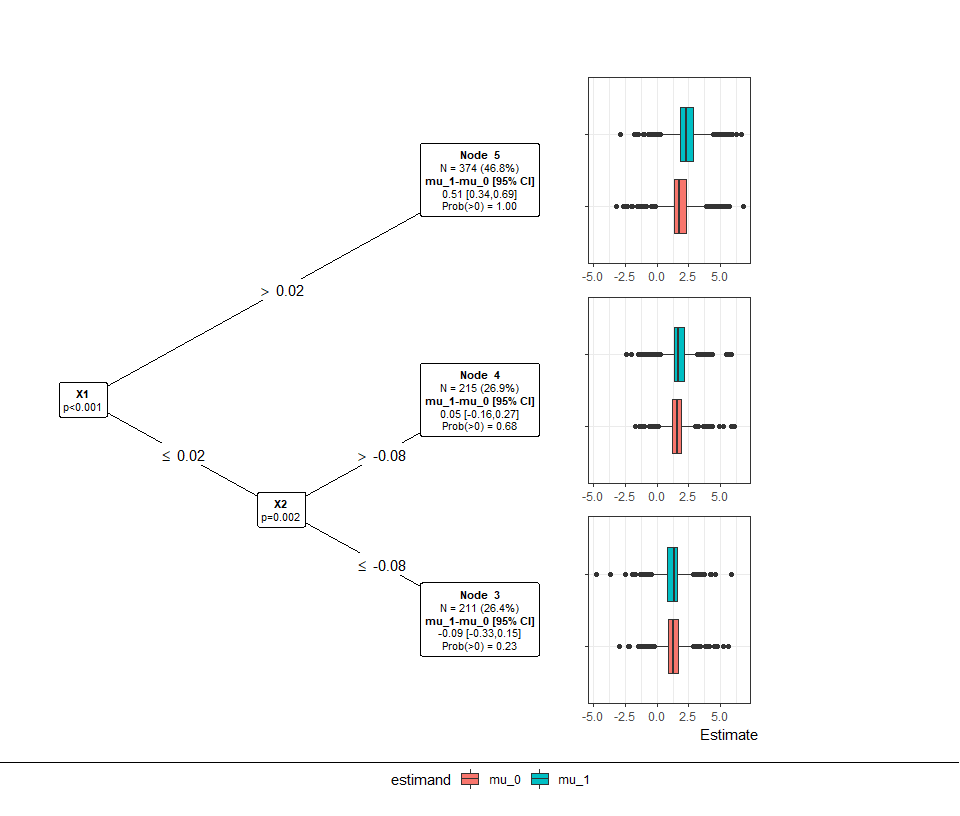# Example: Binary Outcome with Binary Treatment Consider a binary outcome (ex: % overall response rate) with a binary treatment (study drug vs standard of care). The estimand of interest is the risk difference, $$\theta_0 = E(Y|A=1)-E(Y|A=0)$$. Similar to the continous example, we simulate binomial data where roughly 30% of the patients receive no treatment-benefit for using $$A=1$$ vs $$A=0$$. Responders vs non-responders are defined by the continuous predictive covariates $$X_1$$ and $$X_2$$ for a total of four subgroups. Subgroup treatment effects are: $$\theta_{1} = 0$$ ($$X_1 \leq 0, X_2 \leq 0$$), $$\theta_{2} = 0.11 (X_1 > 0, X_2 \leq 0)$$, $$\theta_{3} = 0.21 (X_1 \leq 0, X2 > 0$$), $$\theta_{4} = 0.31 (X_1>0, X_2>0)$$. For binary outcomes (Y=0,1), the default settings are: filter=“glmnet”, ple=“ranger”, submod=“glmtree”" (GLM MOB with identity link), and param=“dr”. dat_bin = generate_subgrp_data(family="binomial", seed = 5558) Y = dat_bin$Y
X = dat_bin$X # 50 covariates, 46 are noise variables, X1 and X2 are truly predictive A = dat_bin$A # binary treatment, 1:1 randomized

res0 = PRISM(Y=Y, A=A, X=X)
#> Observed Data
#> Filtering: glmnet
#> PLE: ranger
#> Subgroup Identification: glmtree
#> Parameter Estimation: dr
summary(res0)
#> $PRISM Configuration #>  "glmnet => ranger => glmtree => dr" #> #>$Variables that Pass Filter
#>   "X1"  "X2"  "X3"  "X5"  "X7"  "X9"  "X15" "X16" "X17" "X19" "X21" "X28"
#>  "X31" "X34" "X35" "X38" "X45"
#>
#> $Number of Identified Subgroups #>  5 #> #>$Variables that Define the Subgroups
#>  "X1, X2, X5, X3"
#>
#> \$Parameter Estimates
#>    Subgrps   N  estimand     est     SE alpha               CI
#> 4        4  86      mu_0  0.0400 0.0307  0.05   [-0.021,0.101]
#> 7        5 199      mu_0  0.1693 0.0312  0.05  [0.1076,0.2309]
#> 10       6 156      mu_0  0.3933 0.0449  0.05   [0.3046,0.482]
#> 13       8 128      mu_0  0.2793 0.0501  0.05  [0.1802,0.3785]
#> 16       9 231      mu_0  0.5630 0.0407  0.05  [0.4828,0.6432]
#> 1     ovrl 800      mu_0  0.3304 0.0198  0.05  [0.2916,0.3692]
#> 5        4  86      mu_1  0.0095 0.0889  0.05 [-0.1672,0.1862]
#> 8        5 199      mu_1  0.3360 0.0622  0.05  [0.2133,0.4587]
#> 11       6 156      mu_1  0.4279 0.0717  0.05  [0.2863,0.5694]
#> 14       8 128      mu_1  0.6565 0.0712  0.05  [0.5155,0.7974]
#> 17       9 231      mu_1  0.7235 0.0562  0.05  [0.6127,0.8342]
#> 2     ovrl 800      mu_1  0.4820 0.0314  0.05  [0.4204,0.5435]
#> 6        4  86 mu_1-mu_0 -0.0305 0.1049  0.05 [-0.2391,0.1781]
#> 9        5 199 mu_1-mu_0  0.1668 0.0695  0.05  [0.0298,0.3038]
#> 12       6 156 mu_1-mu_0  0.0345 0.0836  0.05 [-0.1305,0.1996]
#> 15       8 128 mu_1-mu_0  0.3771 0.0854  0.05  [0.2081,0.5461]
#> 18       9 231 mu_1-mu_0  0.1605 0.0709  0.05  [0.0208,0.3002]
#> 3     ovrl 800 mu_1-mu_0  0.1516 0.0363  0.05  [0.0804,0.2228]
#>
#> attr(,"class")
#>  "summary.PRISM"
plot(res0)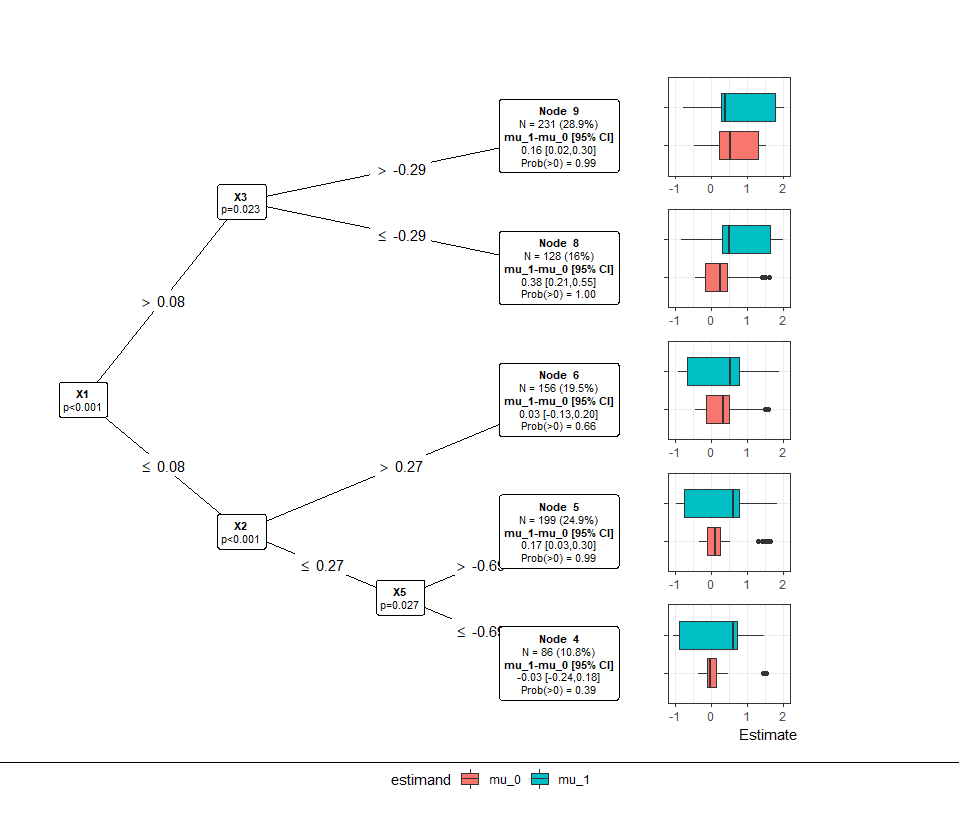# Example: Survival Outcome with Binary Treatment

Survival outcomes are also allowed in PRISM. The default settings use glmnet to filter (“filter_glmnet”), ranger patient-level estimates (“ranger”; for survival, the output is the restricted mean survival time treatment difference), “mob_weib”" (MOB with weibull loss function) for subgroup identification, and param_cox (subgroup-specific cox regression models). Another subgroup option is to use “ctree”", which uses the conditional inference tree (ctree) algorithm to find subgroups; this looks for partitions irrespective of treatment assignment and thus corresponds to finding prognostic effects.

# Load TH.data (no treatment; generate treatment randomly to simulate null effect) ##
data("GBSG2", package = "TH.data")
surv.dat = GBSG2
# Design Matrices ###
Y = with(surv.dat, Surv(time, cens))
X = surv.dat[,!(colnames(surv.dat) %in% c("time", "cens")) ]
set.seed(6345)
A = rbinom(n = dim(X), size=1, prob=0.5)

# Default: glmnet ==> ranger (estimates patient-level RMST(1 vs 0) ==> mob_weib (MOB with Weibull) ==> cox (Cox regression)
res_weib = PRISM(Y=Y, A=A, X=X)
#> Observed Data
#> Filtering: glmnet
#> PLE: ranger
#> Subgroup Identification: mob_weib
#> Parameter Estimation: cox
plot(res_weib, type="PLE:waterfall")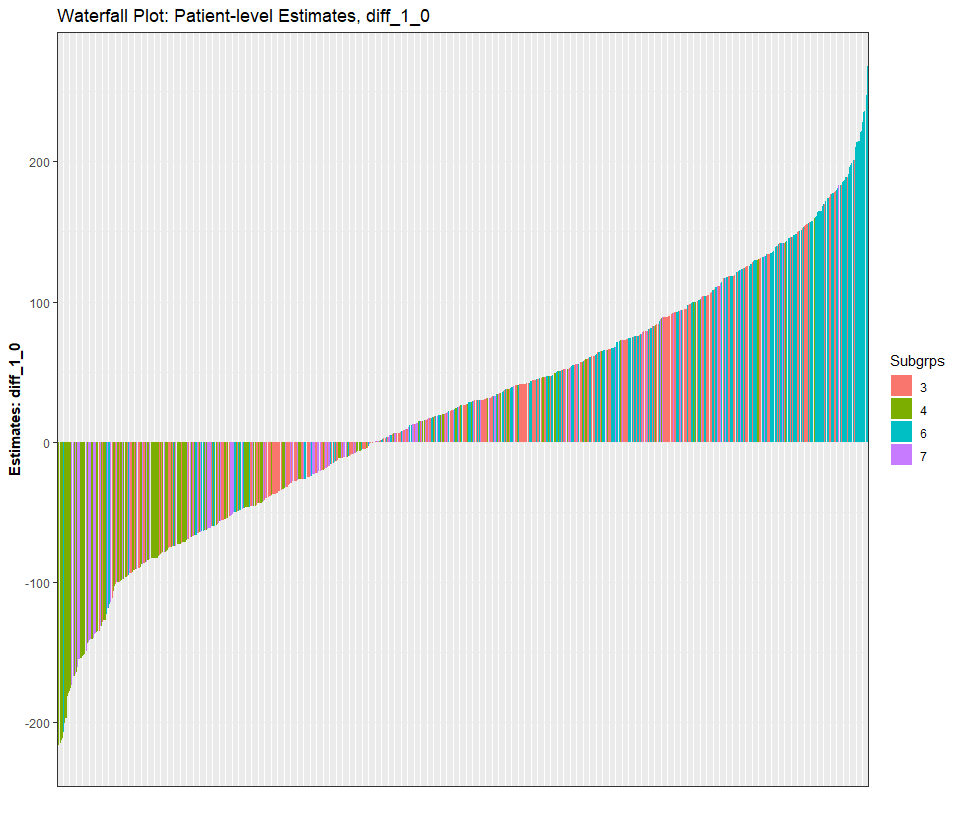plot(res_weib)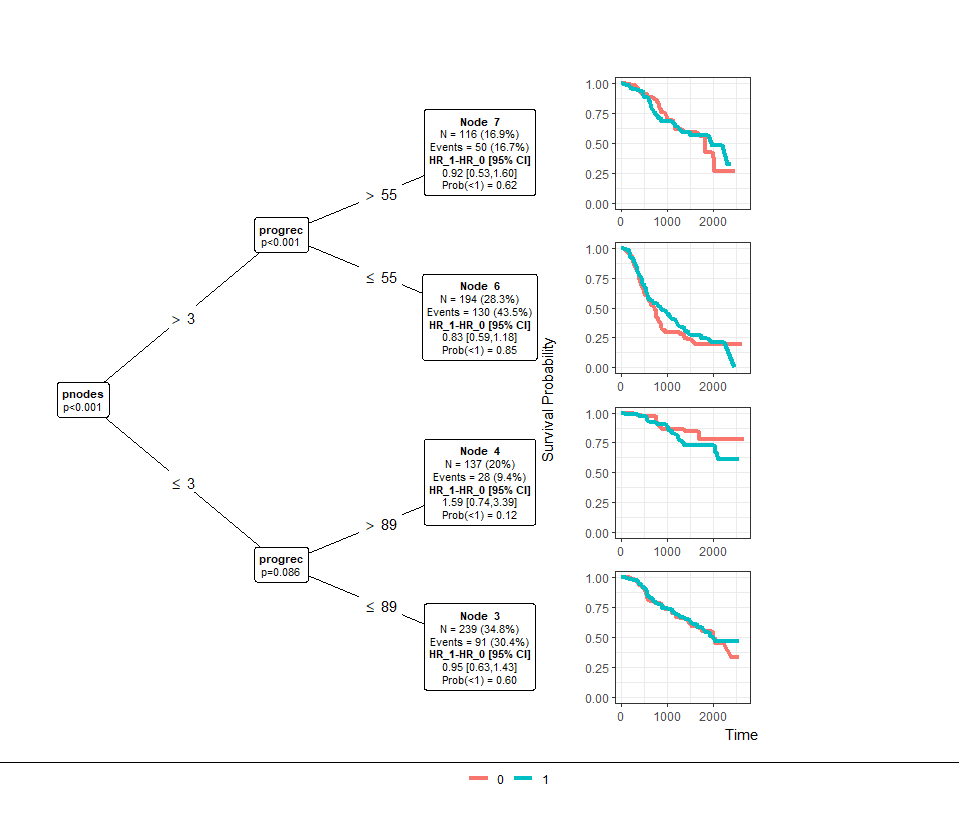# Resampling

Resampling methods are also a feature in PRISM. Bootstrap (resample=“Bootstrap”), permutation (resample=“Permutation”), and cross-validation (resample=“CV”) based-resampling are included. Resampling can be used for obtaining de-biased or “honest” subgroup estimates, inference, and/or probability statements. For each resampling method, the sampling mechanism can be stratified by the discovered subgroups (default: stratify=TRUE). To summarize:

Bootstrap Resampling

Given observed data $$(Y, A, X)$$, fit $$PRISM(Y,A,X)$$. Based on the identified $$k=1,..,K$$ subgroups, output subgroup assignment for each patient. For the overall population $$k=0$$ and each subgroup ($$k=0,...,K$$), store the associated parameter estimates ($$\hat{\theta}_{k}$$). For $$r=1,..,R$$ resamples with replacement ($$(Y_r, A_r, X_r)$$), fit $$PRISM(Y_r, A_r, X_r)$$ and obtain new subgroup assignments $$k_r=1,..,K_r$$ with associated parameter estimates $$\hat{\theta}_{k_r}$$. For subjects $$i$$ within subgroup $$k_r$$, note that everyone has the same assumed point-estimate, i.e., $$\hat{\theta}_{k_r}=\hat{\theta}_{ir}$$. For resample $$r$$, the bootstrap estimates based for the original identified subgroups ($$k=0,...,K$$) are calculated respectively as: $\hat{\theta}_{rk} = \frac{\sum_{i} I(i\in S_k) \hat{\theta}_{ir}}{\sum_{i} I(i\in S_k)}$ The bootstrap smoothed estimate and standard error, as well as probability statements, are calculated as: $\tilde{\theta}_{k} = \frac{1}{R} \sum_r \hat{\theta}_{rk}$ $SE(\hat{\theta}_{k})_B = \sqrt{ \frac{1}{R} \sum_r (\hat{\theta}_{rk}-\tilde{\theta}_{k})^2 }$ $\hat{P}(\hat{\theta}_{k}>c) = \frac{1}{R} \sum_r I(\hat{\theta}_{rk}>c)$ If resample=“Bootstrap”, the default is to use the bootstrap smoothed estimates, $$\tilde{\theta}_{k}$$, along with percentile-based CIs (i.e. 2.5,97.5 quantiles of bootstrap distribution). Bootstrap bias is also calculated, which can be used to assess the bias of the initial subgroup estimates.

Returning to the survival example, we now re-run PRISM with 50 bootstrap resamples (for increased accuracy, use >1000). The smoothed bootstrap estimates, bootstrap standard errors, bootstrap bias, percentile CI, and calibrated CI correspond to “est_resamp”, “SE_resamp”, “bias.boot”, “LCL.pct”/“UCL.pct”, and “LCL.calib”/“UCL.calib” respectively. We can also plot a density plot of the bootstrap distributions through the plot(…,type=“resample”) option.

res_boot = PRISM(Y=Y, A=A, X=X, resample = "Bootstrap", R=50, ple="None")
# Plot of distributions #
plot(res_boot, type="resample", estimand = "HR(A=1 vs A=0)")+geom_vline(xintercept = 1)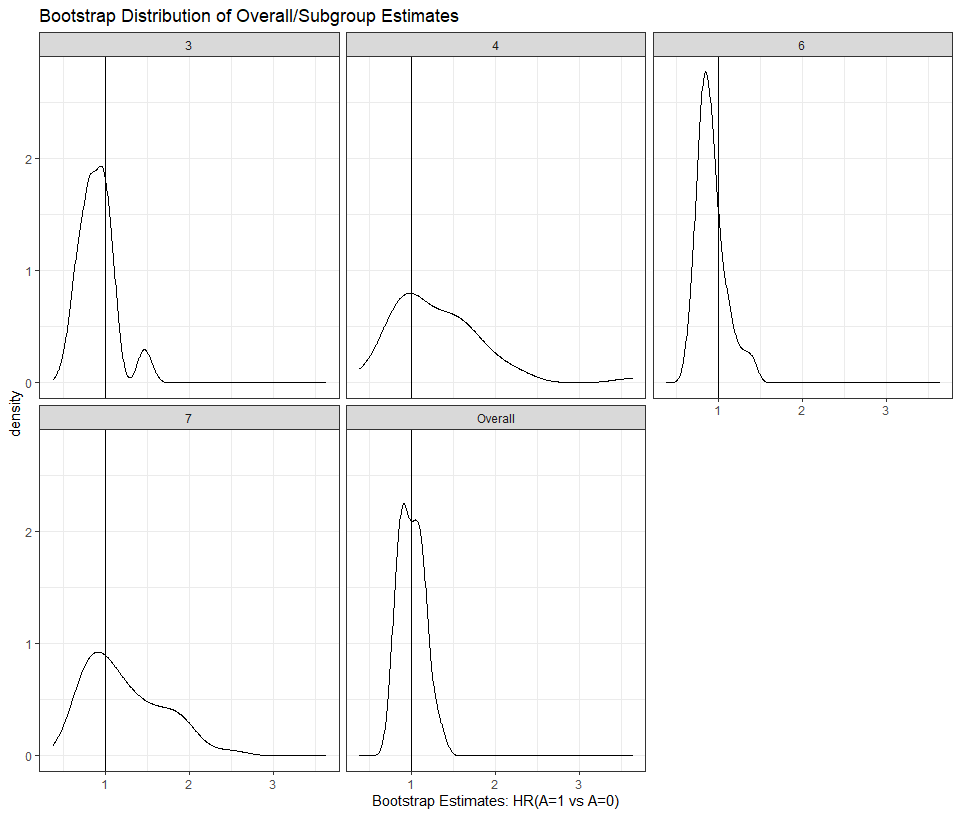Permutation Resampling

Permutation resampling (resample=“Permutation”) follows the same general procedure as bootstrap resampling. The main difference is that we only randomly shuffle the treatment assignment $$A$$ without replacement. This simulates the null hypothesis of no treatment. A key output is the permutation p-values (pval_perm in param.dat) and the permutation resampling distributions.

Cross-Validation

Cross-validation resampling (resample=“CV”) also follows the same general procedure as bootstrap resampling. Given observed data $$(Y, A, X)$$, fit $$PRISM(Y,A,X)$$. Based on the identified $$k=1,..,K$$ subgroups, output subgroup assignment for each patient. Next, split the data into $$R$$ folds (ex: 5). For fold $$r$$ with sample size $$n_r$$, fit PRISM on $$(Y[-r],A[-r], X[-r])$$ and predict the patient-level estimates and subgroup assignments ($$k_r=1,...,K_r$$) for patients in fold $$r$$. The data in fold $$r$$ is then used to obtain parameter estimates for each subgroup, $$\hat{\theta}_{k_r}$$. For fold $$r$$, estimates and SEs for the original subgroups ($$k=1,...,K$$) are then obtained using the same formula as with bootstrap resampling, again, denoted as ($$\hat{\theta}_{rk}$$, $$SE(\hat{\theta}_{rk})$$). This is repeated for each fold and “CV” estimates and SEs are calculated for each identified subgroup. Let $$w_r = n_r / \sum_r n_r$$, then:

$\hat{\theta}_{k,CV} = \sum w_r * \hat{\theta}_{rk}$ $SE(\hat{\theta}_k)_{CV} = \sqrt{ \sum_{r} w_{r}^2 SE(\hat{\theta}_{rk})^2 }$ CV-based confidence intervals can then be formed, $$\left[\hat{\theta}_{k,CV} \pm 1.96*SE(\hat{\theta}_k)_{CV} \right]$$.

# Conclusion

Overall, the StratifiedMedicine package contains a variety of tools (“filter_train”, “ple_train”, “submod_train”, and “PRISM”) and plotting features (“plot_dependence”, “plot_importance”, “plot_ple”) for exploration of hetergeneous treatment effects. Each step is customizable, allowing for fast experimentation and improvement of individual steps. More details on creating user-specific models can be found in the "User_Specific_Models_PRIS vignette User_Specific_Models. The StratifiedMedicine R package will be continually updated and improved.